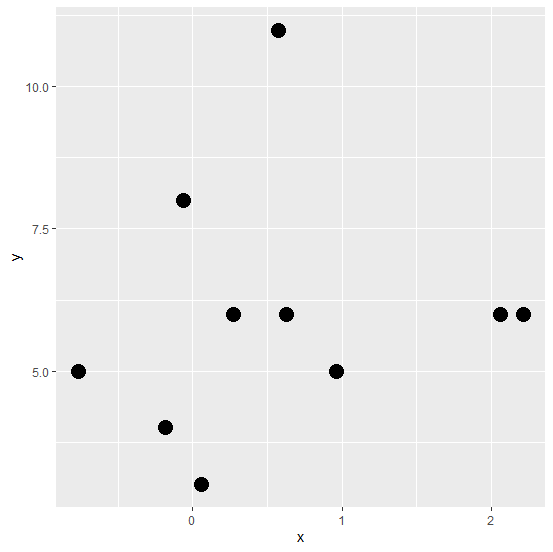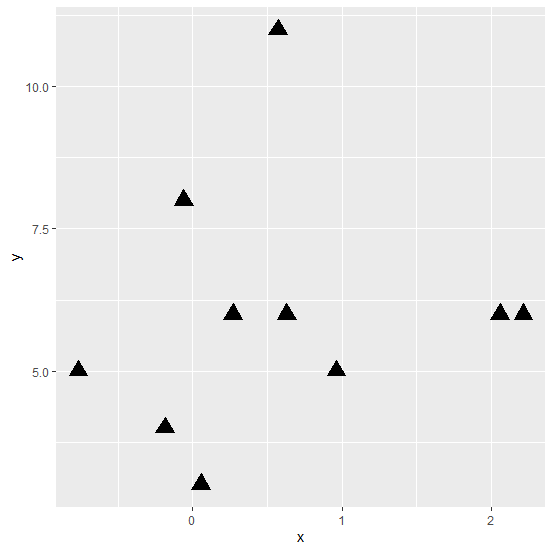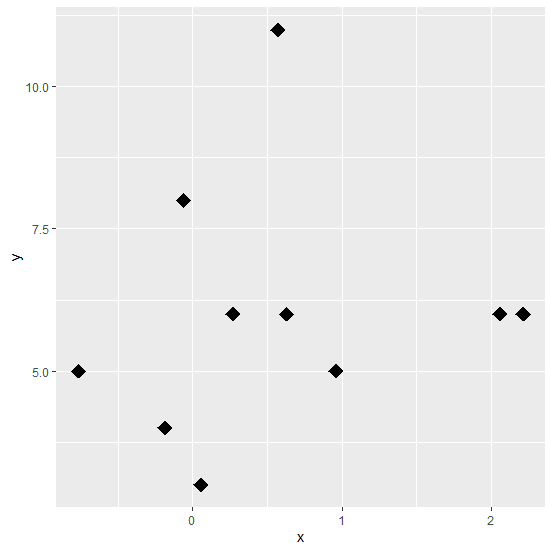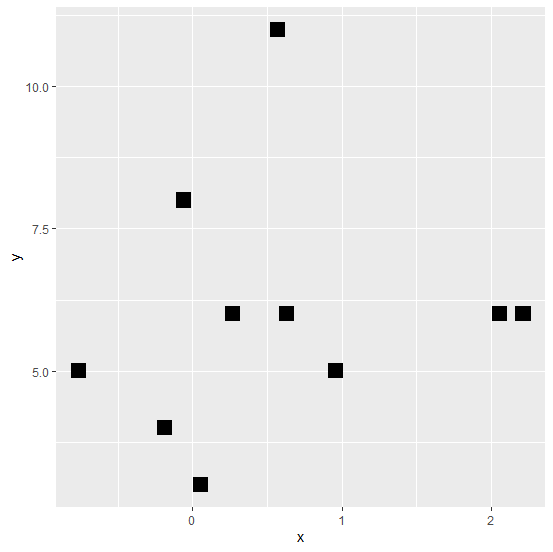# How to create a scatterplot in R using ggplot2 with different designs of points?

Scatterplot helps us to identify the linear relationship between two variables and it is the first step of determining a predictive model. Before using any predictive modeling technique we must draw a scatterplot between independent and dependent variables to check what kind of relationship exists between them. A scatterplot generally represented by circular points on the plot area but we can have different types of points such as square, rectangle, diamond, etc. In ggplot2, pch argument of geom_point can help us to create scatterplot with these types of points.

## Example

Consider the below data frame −

set.seed(123)
x <-rnorm(10,0.5)
y <-rpois(10,5)
df <-data.frame(x,y)
library(ggplot2)

Creating the scatterplot with circular points −

ggplot(df,aes(x,y))+geom_point(pch=16,size=5)

## OutputCreating the scatterplot with triangular points

ggplot(df,aes(x,y))+geom_point(pch=17,size=5)

## OutputCreating the scatterplot with diamond points −

ggplot(df,aes(x,y))+geom_point(pch=18,size=5)


## OutputCreating the scatterplot with square points −

ggplot(df,aes(x,y))+geom_point(pch=15,size=5)

## Output